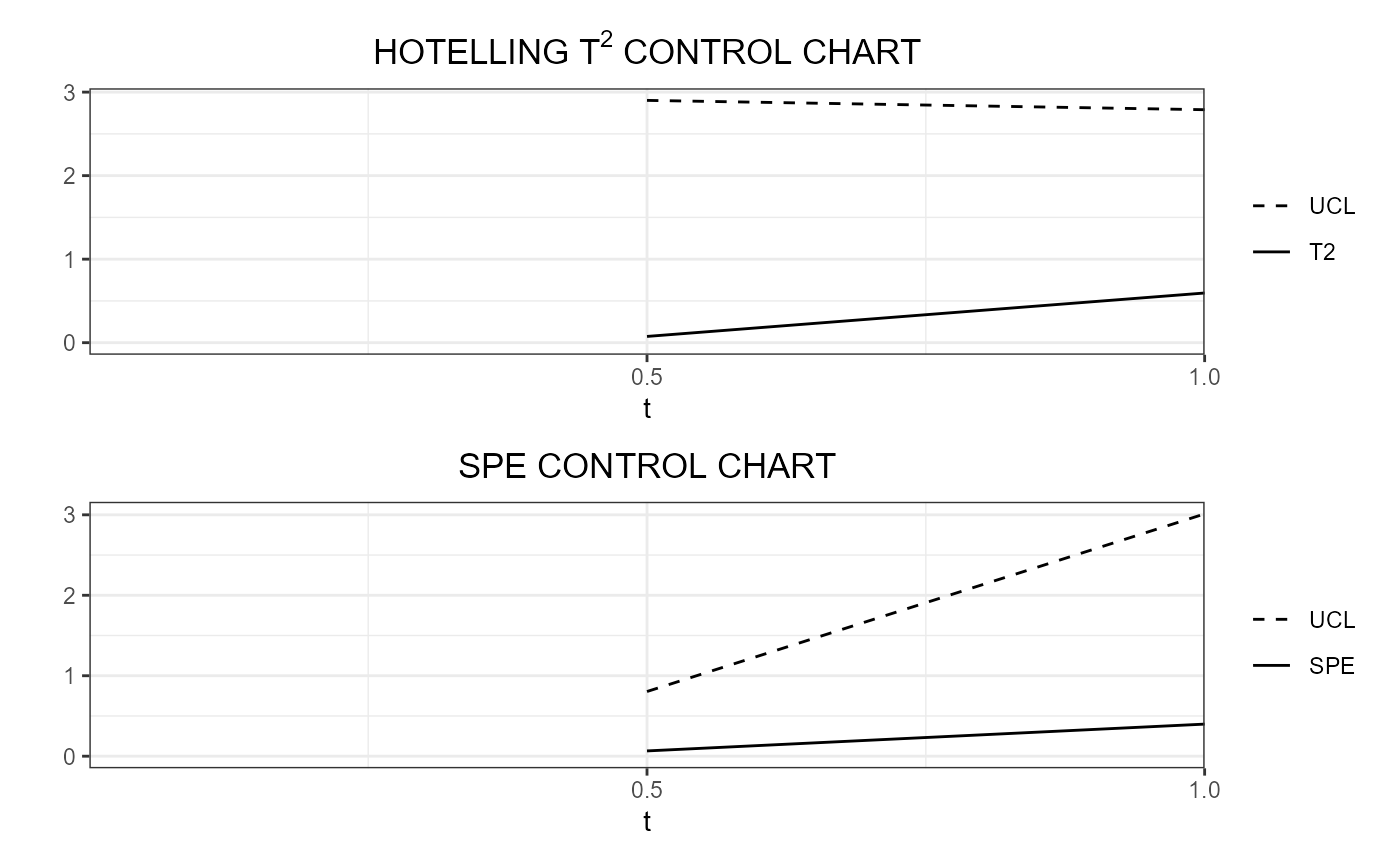This function produces a list of data frames, each of them is produced by control_charts_pca and is needed to plot control charts for monitoring multivariate functional covariates each evolving up to an intermediate domain point.

control_charts_pca_mfd_real_time(
pca_list,
components_list = NULL,
mfdobj_x_test,
mfdobj_x_tuning = NULL,
alpha = 0.05,
limits = "standard",
seed,
nfold = NULL,
tot_variance_explained = 0.9,
single_min_variance_explained = 0,
absolute_error = FALSE,
ncores = 1
)

## Arguments

pca_list

A list of lists produced by pca_mfd_real_time, containing a list of multivariate functional principal component analysis models estimated on functional data each evolving up to an intermediate domain point.

components_list

A list of components given as input to pca_mfd for each intermediate domain point.

mfdobj_x_test

A list created using get_mfd_df_real_time or get_mfd_list_real_time, denoting a list of functional data objects in the phase II monitoring data set, each evolving up to an intermediate domain point, with observations of the multivariate functional data. The length of this list and pca_list must be equal, and their elements in the same position in the list must correspond to the same intermediate domain point.

mfdobj_x_tuning

A list created using get_mfd_df_real_time or get_mfd_list_real_time, denoting a list of functional data objects in the tuning data set (used to estimate control chart limits), each evolving up to an intermediate domain point, with observations of the multivariate functional data The length of this list and pca_list must be equal, and their elements in the same position in the list must correspond to the same intermediate domain point. If NULL, the training data, i.e. the functional data in pca_list, are also used as the tuning data set. Default is NULL.

alpha

See control_charts_pca.

limits

See control_charts_pca.

seed

Deprecated: See control_charts_pca.

nfold

See control_charts_pca.

tot_variance_explained

See control_charts_pca.

single_min_variance_explained

See control_charts_pca.

absolute_error

See control_charts_pca.

ncores

If you want parallelization, give the number of cores/threads to be used when creating objects separately for different instants.

## Value

A list of data.frames each produced by control_charts_pca, corresponding to a given instant.

pca_mfd_real_time, control_charts_pca

## Examples

library(funcharts)
data("air")
air1 <- lapply(air, function(x) x[1:8, , drop = FALSE])
air2 <- lapply(air, function(x) x[9:10, , drop = FALSE])
mfdobj_x1_list <- get_mfd_list_real_time(air1[c("CO", "temperature")],
n_basis = 15,
lambda = 1e-2,
k_seq = c(0.5, 1))
mfdobj_x2_list <- get_mfd_list_real_time(air2[c("CO", "temperature")],
n_basis = 15,
lambda = 1e-2,
k_seq = c(0.5, 1))
pca_list <- pca_mfd_real_time(mfdobj_x1_list)

cclist <- control_charts_pca_mfd_real_time(
pca_list = pca_list,
components_list = 1:3,
mfdobj_x_test = mfdobj_x2_list)
plot_control_charts_real_time(cclist, 1)